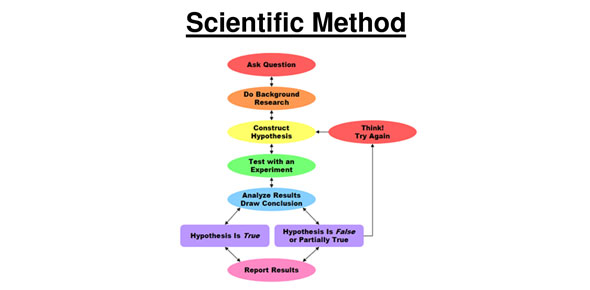Scientific Method & Metric System

25 Questions | Total Attempts: 251SettingsS. M. & Metric Measurements

• 1.
A series of logical steps that is followed in order to solve a problem is called the __________
• A.

Scientific method

• B.

Experimental Process

• C.

Scientific Theory

• D.

Model Method

• 2.
Which of the following steps to solve a problem must be completed first _____________________
• A.

Analyze Data

• B.

State a Hypothesis

• C.

Identify the Problem

• D.

Conduct Experiment

• 3.
A factor in an experiment that can change is a(n)______________
• A.

Variable

• B.

Observation

• C.

Control

• D.

Hypothesis

• 4.
A good way to organize and record your experiment is using a ________
• A.

Calculator

• B.

Data Table

• C.

Hypothesis

• D.

Spring Scale

• 5.
What does a Pascal measure ________?
• A.

Pressure

• B.

Solids

• C.

Liquid

• D.

Air

• 6.
A meter measures ________________?
• A.

Liquids

• B.

Length

• C.

Volume

• D.

Solid

• 7.
The Joule is part of the metric system?
• A.

True

• B.

False

• 8.
What does a gram measure?
• A.

Distance

• B.

Pressure

• C.

Wieght

• D.

Energy

• 9.
How can you abbreviate millilmeters?
• A.

Mm

• B.

Dag

• C.

Cm

• D.

Ms

• 10.
How can you abbreviate milliigrams?
• A.

G

• B.

MG

• C.

Mg

• D.

Cg

• 11.
How can you abbreviate dekagram?
• A.

Dag

• B.

Dg

• C.

Dg

• D.

G

• 12.
How much is a Liter worth in the metric system?
• A.

1

• B.

100

• C.

10

• D.

1000

• 13.
How much is a centi worth in the metric system?
• A.

.1

• B.

.01

• C.

1.0

• D.

100

• 14.
How much is a hecto worth in the metric system?
• A.

10

• B.

100

• C.

1000

• D.

1.0

• 15.
If snails move faster on smoother plane, then a snail will move faster on glass than on sand.
• A.

Hypothesis

• B.

Experiment

• C.

Data

• D.

Conclusion

• 16.
What makes the pupil in the eye change size?
• A.

Experiement

• B.

Hypothesis

• C.

Question

• D.

Conclusion

• 17.
Which color lights cause plants to grow more effectively?
• A.

Conclusion

• B.

Data

• C.

Hypothesis

• D.

Question

• 18.
28 mL = ______ cL
• A.

2.8

• B.

.28

• C.

28

• D.

.028

• 19.
8000dam = _______km
• A.

.8

• B.

80

• C.

8

• D.

80000

• 20.
Which system of measurement is easier?
• A.

Customary

• B.

Metric

• C.

Galactic

• D.

Empire

• 21.
What is the difference between a hypothesis and theory?
• A.

"Theory" is another word for "fact", hypothesis is another word for guess

• B.

Hypothesis can't be proven; theories can

• C.

Theories have been confirmed through tests; hypothesis haven't

• D.

Theories contain many hypotheses; a hypothesis only contains one theory

• 22.
What might cause a theory to change over time?
• A.

New laws passed by the government

• B.

New but untestable ideas

• C.

Changes in public opinion

• D.

The discovery of new evidence

• 23.
Which units measure the same basic quantities?
• A.

Gallons and Liters

• B.

Gallons and Kilograms

• C.

Ounces and centimeters

• D.

Yards and feet

• 24.
If a recipe calls for 3 and 1/4 cups of flour, you can tell that it's using:
• A.

The customary system

• B.

The metric system

• C.

Both the customary system and the metric system

• D.

Neither the customary nor the metric system

• 25.
Quantitative means to express something as a number.
• A.

True

• B.

False

Related TopicsBack to top